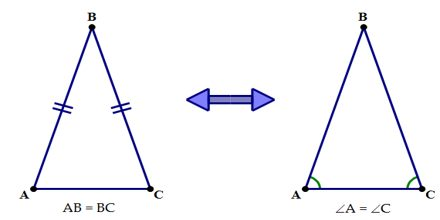Mathematic

# Isosceles Triangle TheoremPrime purpose of this lecture is to present on Isosceles Triangle Theorem. An isosceles triangle is a triangle with (at least) two equal sides. If two sides of a triangle are congruent, then the angles opposite those sides are congruent. The converse of the base angles theorem, states that if two angles of a triangle are congruent, then sides opposite those angles are congruent. An equilateral triangle is a triangle with all three sides of equal length.